#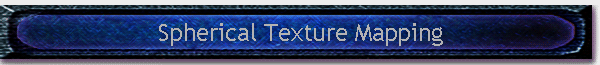Written by Robert Dunlop Microsoft DirectX MVP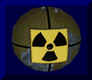When loading a mesh from a modeling package, texture mapping coordinates are usually included to indicate how a texture is applied to the mesh.  However, when generating our own meshes, it will often be necessary to create texture coordinates based on the shape of the object.  One such method for  generating texture coordinates from geometry is known as spherical mapping.

The basis of this mapping technique is to treat the object as if it were a sphere, and mapping coordinates based by determining the position on a sphere by angular displacement.  In this article we'll take a look at two forms of spherical mapping, one based on normals and another based on vertex position.

## Spherical Mapping with Normals

The simplest method is to use the normal of each vertex, which is usually already pre-calculated for the purpose of lighting.  Texture coordinates are generated based on the angle of the surface at each point.  Values for the texture coordinates are calculated as follows: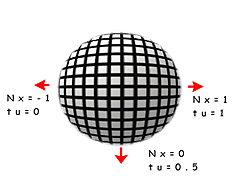The U coordinate is calculated from the X coordinate of the normal, which will vary from -1.0 to 1.0, representing the longitude of a corresponding point on a sphere.  The value is calculated as follows:

## tu = asin(Nx)/PI + 0.5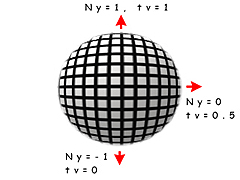The V coordinate is calculated from the Y coordinate of the normal, which will vary from -1.0 to 1.0, representing the latitude of a corresponding point on a sphere.  The value is calculated as follows:

## tv = asin(Ny)/PI + 0.5

As a faster alternative the following equations can be used:

tu = Nx/2 + 0.5
tv = Ny/2 + 0.5

The result is that the coordinates are non-linear, tending to stretch the texture around the Y and Z poles while compressing it around the X poles.

Below is an example of how to implement sphere mapping on a sphere generated with D3DX:

struct _VERTEX
{
D3DXVECTOR3 pos;     // vertex position
D3DXVECTOR3 norm;    // vertex normal
float tu;            // texture coordinates
float tv;
} VERTEX,*LPVERTEX;

#define FVF_VERTEX    D3DFVF_XYZ|D3DFVF_NORMAL|D3DFVF_TEX1

LPD3DXMESH CreateMappedSphere(LPDIRECT3DDEVICE8 pDev,float fRad,UINT slices,UINT stacks)
{
// create the sphere
LPD3DXMESH mesh;
return NULL;

// create a copy of the mesh with texture coordinates,
// since the D3DX function doesn't include them
LPD3DXMESH texMesh;
if (FAILED(mesh->CloneMeshFVF(D3DXMESH_SYSTEMMEM,FVF_VERTEX,pDev,&texMesh)))
// failed, return un-textured mesh
return mesh;

// finished with the original mesh, release it
mesh->Release();

// lock the vertex buffer
LPVERTEX pVerts;
if (SUCCEEDED(texMesh->LockVertexBuffer(0,(BYTE **) &pVerts))) {

// get vertex count
int numVerts=texMesh->GetNumVertices();

// loop through the vertices
for (int i=0;i<numVerts;i++) {

// calculate texture coordinates
pVerts->tu=asinf(pVerts->norm.x)/D3DX_PI+0.5f;
pVerts->tv=asinf(pVerts->norm.y)/D3DX_PI+0.5f;

// go to next vertex
pVerts++;
}

// unlock the vertex buffer
texMesh->UnlockVertexBuffer();
}

// return pointer to caller
return texMesh;
}

## Positional Spherical MappingIn some cases, using normals to calculate spherical texture coordinates may not be appropriate.  For example, objects which use face normals, such as the cube illustrated on the right, would have the same coordinates applied to each vertex of a face.  Also, regions that are relatively flat on one or more axis will cause the texture to appear stretched.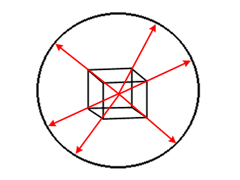An alternative to this is to project a vector from the center of the object outward through each vertex, and use the normalized X and  Y coordinates to calculate the texture coordinates.  This has the effect of projecting each vertex onto a theoretical sphere.  An example of this technique is shown in the following code snippet:

// determine extents
D3DXVECTOR3 vMin,vMax;
D3DXComputeBoundingBox(pVerts,numVerts,FVF_VERTEX,&vMin,&vMax);

// calculate center
D3DXVECTOR3 vCent;
vCent=(vMax+vMin)*0.5f;

// loop through the vertices
for (i=0;i<numVerts;i++) {

// calculate normalized offset from center
D3DXVECTOR3 v;
v=pVerts->pos-vCent;
D3DXVec3Normalize(&v,&v);

// calculate texture coordinates
pVerts->tu=asinf(v.x)/D3DX_PI+0.5f;
pVerts->tv=asinf(v.y)/D3DX_PI+0.5f;

// go to next vertex
pVerts++;
}

Back to The TopThis site, created by DirectX MVP Robert Dunlop and aided by the work of other volunteers, provides a free on-line resource for DirectX programmers.

Special thanks to WWW.MVPS.ORG, for providing a permanent home for this site.

#####Visitors Since 1/1/2000:Last updated: 07/26/05.